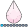## Highlighting in a correlation matrix

Hello,

I am currebtly running some analyses on a data set of mine. In doing so, I am creating a correlation matrix of all the relevant variables (to examine descriptives). I have about 80 variables.

I would like to have SAS highlight the cells in the correlation matrix that have a p-value less than 0.05 (since I have so many variables, it is really hard to see all the correlations that are less than 0.05).

I would love to have the matrix (along with the highligting) outputted to a pdf.

I am fairly new to SAS. So far, I have the matrix and I have it outputted to a pdf, but I cannot seem to figure out how to highlight the cells conditionally within a procedure step.

Can anyone help me? Is it even possible?

Thank you for any help!

9 REPLIES 9art297
Opal | Level 21

## Highlighting in a correlation matrix

Hi Art,

Thank you so much!  That was very helpful!

I do have one question. Currently, with the code included on that link, SAS highlights based on the correlation value. Do you know if it is possible to highlight based on the p-value? I have not been able to find an option to use to specify that with.

But again, thank you so much! :smileygrin:

Camilladata_null__

## Highlighting in a correlation matrix

This is crude but you should be able to adapt to your needs.

proc template;

edit Base.Corr.StackedMatrix;

column (RowName RowLabel) (Matrix) * (Matrix2) * (Matrix3);

edit matrix2;

cellstyle

_val_  = ._ as {backgroundcolor=CXEEEEEE},

_val_  <= 0.0001 as {backgroundcolor=red};

/*                      _val_ <= -0.75 as {backgroundcolor=red},*/

/*                      _val_ <= -0.50 as {backgroundcolor=blue},*/

/*                      _val_ <= -0.25 as {backgroundcolor=cyan},*/

/*                      _val_ <=  0.25 as {backgroundcolor=white},*/

/*                      _val_ <=  0.50 as {backgroundcolor=cyan},*/

/*                      _val_ <=  0.75 as {backgroundcolor=blue},*/

/*                      _val_ <   1.00 as {backgroundcolor=red},*/

/*                      _val_  =  1.00 as {backgroundcolor=CXEEEEEE};*/

end;

end;

run;

ods html body='corr.html' style=statistical;

ods listing close;

proc corr data=sashelp.cars;

ods select PearsonCorr;

run;

ods listing;

ods html close;

proc template;

delete Base.Corr.StackedMatrix;

run;

## Highlighting in a correlation matrix

That did help, thank you!

One more question (I know, I keep asking).

Here is my code:

proc template;

edit Base.Corr.StackedMatrix;

column (RowName RowLabel) (Matrix) * (Matrix2) * (Matrix3);

edit matrix2;

cellstyle

_val_  = ._ as {backgroundcolor=CXEEEEEE},

_val_  <= 0.10 as {backgroundcolor=lime},

_val_  <= 0.05 as {backgroundcolor=lilac},

_val_  <=  0.01 as {backgroundcolor=steel};

end;

end;

run;

/****/

ods html body='corrwolhighlight.html' style=statistical;

ods listing close;

proc corr data=work.wol;

ods select PearsonCorr;

run;

ods listing;

ods html close;

proc template;

delete Base.Corr.StackedMatrix;

run;

I attempted to do mulitple color/background statements (as seen above), however, in my output, I can only get the lime green out. I think SAS overrides the two latter background statements, because they are essentially included in the first statement (<= 0.1 insludes 0.05 and 0.01). The example code was able to specify multiple colors. How can I edit my code to make sas not override the latter two background statements?

Thank you all so much, you have been extremely helpful!Tim_SAS
Barite | Level 11

## Re: Highlighting in a correlation matrix

SAS evaluates the expressions from left to right. As soon as one of the expressions is true, SAS uses that style element and doesn't evaluate any remaining expressions. Put your tests in the order of most restrictive to less restrictive, that is, put _val_ = ._ first, then _val_ <= 0.01, then _val_ <= 0.05, etc.

http://support.sas.com/documentation/cdl/en/odsug/61723/HTML/default/viewer.htm#a001023977.htm

## Highlighting in a correlation matrix

Oh of course!!! I figured that was waht it was doing, but apparently I did not have the brain power to think to reverse list my statements. THANK YOU!! :smileygrin:data_null__

## Highlighting in a correlation matrix

change the order .01, .05, .10

## Re: Highlighting in a correlation matrix

Hello,

I am trying to do the same thing - color code the cell background color based on numbers but I am having difficulties getting an output.

I have 107 variables named snpre_1 snpre_2.... snpre_107 and it is an ordinal scale (1-5) and none of the variables are normally distributed. Therefore, I am using Spearman's correlation. There are no errors but when I go to the specified file (C:\Users\jkim578...), I do not see any files.

What is the "Base.corr.stackedmatrix" referring to? There is an error message saying "Warning: 'Base.Corr.stackedamtrix' does not exist!"

Here is my code:

``````PROC TEMPLATE;
EDIT BASE.CORR.STACKEDMATRIX;
COLUMN (SNPRE_1-SNPRE_107)(Matrix)(Matrix2);
EDIT MATRIX;
CELLSTYLE 	_VAL_ = -1.00 as {background=yellow},
_VAL_ <=-0.40 AS {BACKGROUND=YELLOW},
_VAL_ <= 0.40 AS {BACKGROUND=RED},
_VAL_ <1.00 AS {BACKGROUND=WHITE},
_VAL_ = 1.00 AS {BACKGROUND=WHITE};
END;
END;
RUN;

ODS PDF FILE="C:\Users\jkim578\Documents\corr1.PDF" STYLE=STATISTICAL;
ODS LISTING CLOSE;
PROC CORR spearman DATA=ifsns NOPROB;
VAR SNPRE_1-SNPRE_107;
ODS SELECT SpearmanCorr;
RUN;

ODS LISTING;
ODS HTML CLOSE;

PROC TEMPLATE;
DELETE BASE.CORR.STACKEDMATRIX;
RUN;
``````

## Re: Highlighting in a correlation matrix

Nevermind about my problem! I restarted SAS and just ran my data file and the code below and it worked. All i needed to do was change the file name.

proc template;
edit Base.Corr.StackedMatrix;
column (RowName RowLabel) (Matrix) * (Matrix2);
edit matrix;
cellstyle _val_ = -1.00 as {backgroundcolor=CXEEEEEE},
_val_ <= -0.75 as {backgroundcolor=red},
_val_ <= -0.50 as {backgroundcolor=blue},
_val_ <= -0.25 as {backgroundcolor=cyan},
_val_ <= 0.25 as {backgroundcolor=white},
_val_ <= 0.50 as {backgroundcolor=cyan},
_val_ <= 0.75 as {backgroundcolor=blue},
_val_ < 1.00 as {backgroundcolor=red},
_val_ = 1.00 as {backgroundcolor=CXEEEEEE};
end;
end;
run;

ods html body='corr.html' style=statistical;
ods listing close;
proc corr data=ifsns noprob;
ods select PearsonCorr;
run;
ods listing;
ods html close;

proc template;
delete Base.Corr.StackedMatrix;
run;

Discussion stats
• 9 replies
• 3872 views
• 4 likes
• 5 in conversation